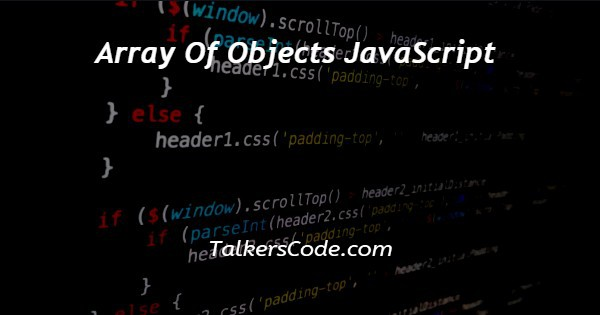# Array Of Objects JavaScript

Last Updated : Jan 1, 2023In this tutorial we will show you the solution of array of objects JavaScript, and in this series, we are going to discuss how to create, update and use a loop through an array of objects.

After this, we will sort them according to keys, values, and alphabetically also.

There are more things that we will discuss in this session of objects. But today we are going to understand how to convert arrays to objects using JavaScript.

## Step By Step Guide On Array Of Objects JavaScript :-

There are many ways with help of we will convert arrays to objects using JavaScript. The ways are:

• With the help of the object.assign() method
• With the help of the array.foreach() method
• With the help of the array.reduce() method

In this article, we are going to show conversion with help of an object.assign() whereas in the next article we will understand another method.

Below, we will show you an example with the help of codes. Let us understand the example given for quickly learning.

```// using object.assign() method
<script>
const raw = ['Robert, 'steve', 'tom','thanos'];
const result = Object.assign({}, raw);
console.log(result)
</script>```
1. As we here see that we today write only JavaScript codes in our example.
2. In previous tutorials, we already many times create a full code example in which we first create an HTML form with its basic structure. We use HTML, head, title, body, and script tags inside that structure.
3. In this example, as we see only, we write our JavaScript codes. The paragraph tag used here is only for display outputs or we can also use console instead of HTML tags. Now, let us understand our examples one by one.
4. In the first example, we see that a paragraph tag is created with an id. After that, we create our Script tag. A script tag is paired tag and is used to write scripts in between the opening and closing tag.
5. In this article, we are going to show you how to convert an array to an object using JavaScript. As specified there are many ways with help of which we can convert array to object. And in this article, we will show you three main ways that are objects.assign(), array.foreach() and array.reduce().
6. Whereas in this article, we will describe only one way which is with the help of an object.assign() method. So, let us understand the codes given above so that we can learn about object.assign(). In the example given above, as you see we first create an array with some items inside this.
7. After this to convert the array to an object, we use the object.assign(). And use curly braces with the name of the array. After this, we just store the result inside a variable. Now, our last task is to show the result after conversion. So, we just console the output.

## Conclusion :-

At last, in conclusion, here we can say that with the help of this article we can understand how to convert an array to an object using JavaScript.

I hope this tutorial on array of objects JavaScript helps you and the steps and method mentioned above are easy to follow and implement.